Courses

# MCQ : Statistics - 1

## 10 Questions MCQ Test Mathematics (Maths) Class 10 | MCQ : Statistics - 1

Description
This mock test of MCQ : Statistics - 1 for Class 10 helps you for every Class 10 entrance exam. This contains 10 Multiple Choice Questions for Class 10 MCQ : Statistics - 1 (mcq) to study with solutions a complete question bank. The solved questions answers in this MCQ : Statistics - 1 quiz give you a good mix of easy questions and tough questions. Class 10 students definitely take this MCQ : Statistics - 1 exercise for a better result in the exam. You can find other MCQ : Statistics - 1 extra questions, long questions & short questions for Class 10 on EduRev as well by searching above.
QUESTION: 1

Solution:
QUESTION: 2

### di is the deviation of xi from assumed mean a.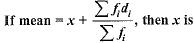Solution: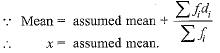QUESTION: 3

### Construction of a cumulative frequency table is useful in determining the

Solution:

A cumulative frequency table helps to determine the median of the series.

QUESTION: 4

Mean of 100 items is 49. It was discovered that three items which should have been 60, 70, 80 were wrongly read as 40, 20, 50 respectively. The correct mean is

Solution:

Sum of 100 observations = 100 x 49 = 4900
Correct sum = 4900 - [40 + 20 + 50] + [60 + 70 + 80] = 5000
∴ Correct mean = 5000/100 = 50

QUESTION: 5

In the formula, Mode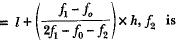Solution:
QUESTION: 6

Choose the correct answer from the given four options : In the formula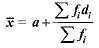for finding the mean of grouped data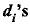are deviation from a of

Solution:
QUESTION: 7

For the following distribution: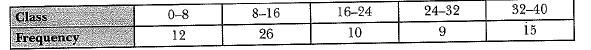The sum of upper limits of the median class and model class is

Solution:
QUESTION: 8

While computing mean of grouped data, we assume that the frequencies are

Solution:
QUESTION: 9

Consider the following frequency distribution of the height of 60 students of a class: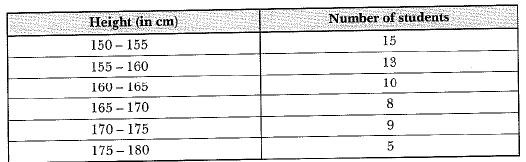The sum of the lower limit of the modal class and upper limit of the median class is

Solution:
QUESTION: 10

For the following distribution: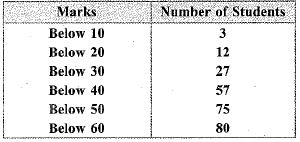the modal class is

Solution: